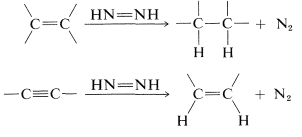# 11.5: Hydrogenation with Diimide

$$\newcommand{\vecs}{\overset { \rightharpoonup} {\mathbf{#1}} }$$ $$\newcommand{\vecd}{\overset{-\!-\!\rightharpoonup}{\vphantom{a}\smash {#1}}}$$$$\newcommand{\id}{\mathrm{id}}$$ $$\newcommand{\Span}{\mathrm{span}}$$ $$\newcommand{\kernel}{\mathrm{null}\,}$$ $$\newcommand{\range}{\mathrm{range}\,}$$ $$\newcommand{\RealPart}{\mathrm{Re}}$$ $$\newcommand{\ImaginaryPart}{\mathrm{Im}}$$ $$\newcommand{\Argument}{\mathrm{Arg}}$$ $$\newcommand{\norm}{\| #1 \|}$$ $$\newcommand{\inner}{\langle #1, #2 \rangle}$$ $$\newcommand{\Span}{\mathrm{span}}$$ $$\newcommand{\id}{\mathrm{id}}$$ $$\newcommand{\Span}{\mathrm{span}}$$ $$\newcommand{\kernel}{\mathrm{null}\,}$$ $$\newcommand{\range}{\mathrm{range}\,}$$ $$\newcommand{\RealPart}{\mathrm{Re}}$$ $$\newcommand{\ImaginaryPart}{\mathrm{Im}}$$ $$\newcommand{\Argument}{\mathrm{Arg}}$$ $$\newcommand{\norm}{\| #1 \|}$$ $$\newcommand{\inner}{\langle #1, #2 \rangle}$$ $$\newcommand{\Span}{\mathrm{span}}$$$$\newcommand{\AA}{\unicode[.8,0]{x212B}}$$

There are alternative ways to add hydrogen to a multiple bond besides the catalytic methods described in the previous sections. The most useful of these are homogeneous reactions utilizing diimide, $$\ce{HN=NH}$$, and diborane, $$\ce{B_2H_6}$$.

The behavior and reactivity of diimide can be understood best by considering the thermochemistry of hydrogenation of nitrogen:The first step is strongly endothermic and is the main hurdle to overcome in the hydrogenation of nitrogen to ammonia. Conversely, the reverse reaction, which is the dehydrogenation of diimide, is strongly exothermic. Therefore we may expect that diimide will have a pronounced tendency to revert to molecular nitrogen. This is in fact so and, at normal temperatures, diimide exists only as a transient intermediate that cannot be isolated. It is extremely reactive and readily transfers hydrogen to carbon-carbon multiple bonds:In practice, diimide is generated as it is needed in the presence of the compound to be hydrogenated. There are various ways to do this, but one of the simplest methods is dehydrogenation of hydrazine with oxidizing agents such as atmospheric oxygen or hydrogen peroxide:

$\ce{H_2N-N_2} \underset{\text{or} \: \ce{O_2}}{\overset{\ce{H_2O_2}}{\longrightarrow}} \ce{HN=NH} + 2 \ce{H}^\oplus + 2 \ce{e}^\ominus$

Hydrazine actually has been used as a hydrogenating agent for over sixty years, but it was not until the 1960's that the diimide intermediate in such reactions was recognized.

The hydrogenation step is stereospecific and transfers hydrogen in the suprafacial manner. For example, alkynes are converted to cis-alkenes:There are no detectable intermediate stages or rearrangements in diimide hydrogenation. The reaction is visualized as a six-center (pericyclic) process in which the bonds are broken and made in a concerted fashion:An important difference between diimide hydrogenation and catalytic hydrogenation is that diimide will react only with symmetrical or nonpolar bonds $$\left( \ce{C=C}, \: \ce{C \equiv C}, \: \ce{N=N} \right)$$, whereas hydrogen can add, albeit reluctantly, to polar bonds $$\left( \ce{C=O}, \: \ce{C=N} \right)$$. Diimide does not attack the stronger polar bonds probably because it does not survive long enough to do so. It selfdestructs in the absence of a reactive substrate to give nitrogen and hydrazine: